# Guest post by Dan Garber and Elad Hazan: The Conditional Gradient Method, A Linear Convergent Algorithm – Part I/II

In a previous post Sebastien presented and analysed the conditional gradient method for minimizing a smooth convex functionover a compact and convex domain. The update step of the method is as follows,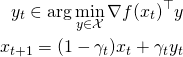where,.

The appeal of the conditional gradient method is two fold:

i) the update step of the method requires only to minimize a linear objective over the domain, which for many domains of interest is computationally cheap (examples are various polytopes that arise in combinatorial optimization such as the flow polytope, the spanning tree polytope and the matching polytope, and the set of unit-trace positive semidefinite matrices), whereas other first order methods require to compute a projection onto the convex set with respect to a certain norm on each iteration which can be computationally much more expensive, and

ii) the method yields sparse solutions in the sense that afteriterations and provided that the first iterate is a vertex of the convex domain, the current iterate is naturally given as the convex sum of at mostvertices of the convex domain (for the simplex this means at mostnon-zero entries and for the set of unit-trace psd matrices this means rank at most).

Ifis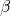-smooth and the diameter ofis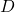(i.e.) then choosingguarantees that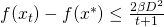. This is also the convergence rate of projected gradient descent for smooth optimization. In case the objective functionis both-smooth and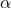-strongly convex (with respect to the same norm) it is known that projected gradient descent has an error that decreases exponentially fast: an additive erroris guaranteed after at most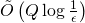iterations whereis the condition number of(the complexity notationis the same as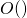but omits factors that are logarithmic in the diameter ofand in). What about the conditional gradient method in this case? So our first question for this post is the following,

Question 1: Is there a CG method that given a-smooth and-strongly convex functionguarantees anadditive error after at mostlinear optimization steps over the domain?

The answer is no, as observed here. In particular the convergence rate of a linearly converging CG method must depend polynomially on the dimension which is not the case for the projected gradient descent method. This brings us to our second question,

Question 2: Is there a CG method that given a-smooth and-strongly convex functionguarantees anadditive error after at mostlinear optimization steps over the domain?

Note that albeit the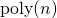factor such a result is still interesting since the time to compute a euclidean projection to the domain (or non-euclidean in case of mirror descent methods) may be longer than the time to minimize a linear objective over the domain by amultiplicative factor.

Here is the place to remark that several linearly converging CG methods for smooth and strongly convex optimization were proposed before but they rely on assumptions regarding the location ofwith respect to the boundary of. For example iflies in the interior of(which means that the problem is an unconstrained one) then the original CG method due to Frank and Wolfe converges exponentially fast, however the number of iterations depends polynomially on the distance offrom the boundary, see this paper by Guélat and Marcotte. In caseis a polytope then a modification of the CG method presented in the same paper, gives a linear convergence rate that is polynomial in the distance offrom the boundary of the smallest facet ofthat contains. Here however we don’t want to rely on such strong assumptions on the location ofand we aim at a linearly converging method that is free from such restrictions.

In the rest of this post we follow our new paper which describes a new CG method for smooth and strongly convex optimization with convergence rate of the form stated in question 2 in caseis a polyhedral set. Such the convergence rate will depend on geometric properties of the set. The latter dependence is very reasonable for many polytopes that arise in combinatorial optimization problems; indeed domains for which fast and simple combinatorial algorithms for linear optimization exists are what in part makes CG methods an attractive approach for non-linear optimization.

To begin the derivation of this new CG method, let us recall that as Sebastien showed here, the conditional gradient method satisfies the following inequality for each iteration,where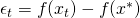.

The fact that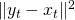might be as large as the diameter ofwhile the approximation error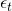may be arbitrarily small forces one to choose step sizes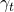that decrease roughly likein order to get the knownconvergence rate.

Let us now consider the case thatis also-strongly convex. That is,In particular the above inequality implies that,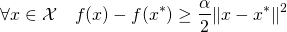Now assume that the iteratesatisfies thatfor some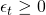and denote by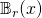the euclidean ball of radiuscentred at point. Define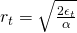and let us now chooseas the optimal solution to the following problem,Note that CG inequality still holds since by the strong convexity we have thatand the only important thing in the selection ofthat is required for the CG inequality to hold is that. We’ll now get that,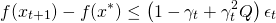Thus choosing a constant step size like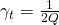and an initial error bound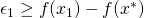results in exponentially fast convergence.

The problem with the above approach is of course that now the optimization problem that needs to be solved on each iteration is no longer a linear minimization step over the original domainbut a much more difficult problem due to the additional constraint on the distance offrom. What we would like to get is an exponentially fast converging method that still requires to solve only a linear minimization problem overon each iteration. This will be the subject of our next post.

This entry was posted in Optimization. Bookmark the permalink.